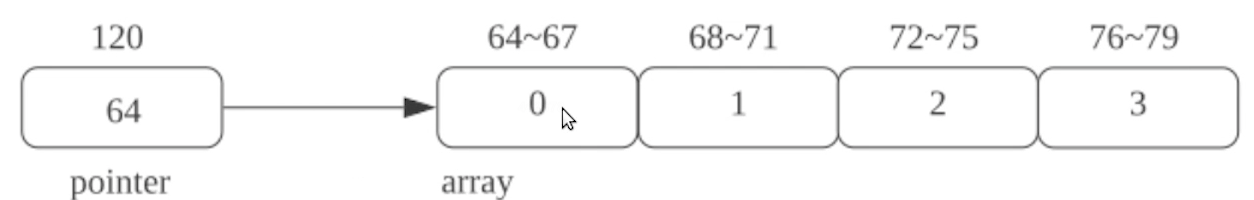# 第四章：数组、vector与字符串（一）

2021/7/21 4:40:29 浏览：

# 数组

## 数组基础

``````int b;			// 类型为int
``````

• 缺省初始化，会将数组中的每一个元素都按照缺省初始化的方式进行初始化，局部变量初始化为随机值，全局变量、线程相关变量和静态变量初始化为0。比如
``````int b;
``````
• 聚合初始化，比如
``````int b = {1,2,3};  //初始化为1，2，3
int b = {1,2};	 //初始化为1，2，0
int b = {};		 //初始化为0，0，0
int b[] = {1,2,3};	 //初始化为1，2，3并自动推导b的类型为int
int b[];			 //不合法的代码
int b = {1,2,3};	 //不合法的代码
``````

• 不能使用auto来声明数组类型
``````auto b = {1,2,3}; 		// b的类型不是int，而是std::initializer_list<int>
``````
• 数组是不能复制的
``````int b = {1,3,4};
int a = b;			// 这里会报错
``````
• 数组的元素个数必须是一个常量表达式（编译期可以计算的值）
• 字符串数组具有一定的特殊性
``````char Str[] = "Hello";				// 类型为char,结尾自动添加了一个'\0'
char Str[] = {"H","e","l","l","o"}; // 类型为char
``````

• 指针数组与数组的指针
``````int * a;				// 指针数组
int (*a);			// 指向数组的指针
``````
• 数组的引用
``````int b;
int (&a) = b;
``````

注意，引用的数组（比如`int & a`）是不合法的

``````	int a = {1,2,3};
a;
a;
a;
``````

• 数组对象是一个左值
• 数组在使用时通常会转换成对应的指针类型，指向数组中第一个元素的地址，比如
``````int a;
auto b = a;				// 这里的b是int*类型，指向a的地址
``````
• 数组访问的形式`x[y]`会被解析为`*((x)+(y))`
• 访问时一定要避免数组下标的越界

## 从数组到指针

``````	int a;
auto b = a;				// 这里的b是int*类型，指向a的地址
``````

``````int a;
auto & b = a;
````````````int a = {1,2,3};
std::begin(a);			// 指向数组第一个元素的指针
std::end(a);			// 指向数组最后一个元素的下一个元素的指针
std::cbegin(a);			// 指向数组第一个元素的指向常量的指针
std::cend(a);			// 指向数组最后一个元素的下一个元素的指向常量的指针
``````

• 增加、减少
• 比较

大于小于的比较只有针对同一个数组才有意义

• 求距离：两个指针相减会先对地址进行相减，之后除以指针指向的类型的尺寸，得到两个指针指向的对象在数组中的距离
• 解引用（`*`
• 指针索引（`[]`